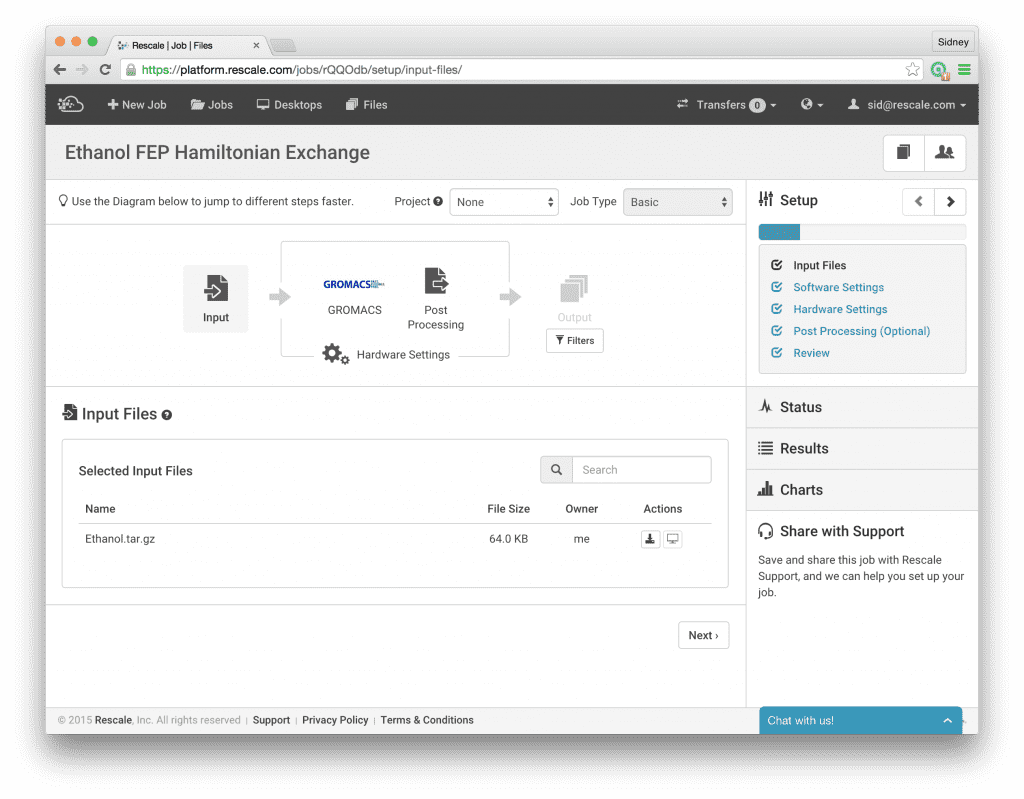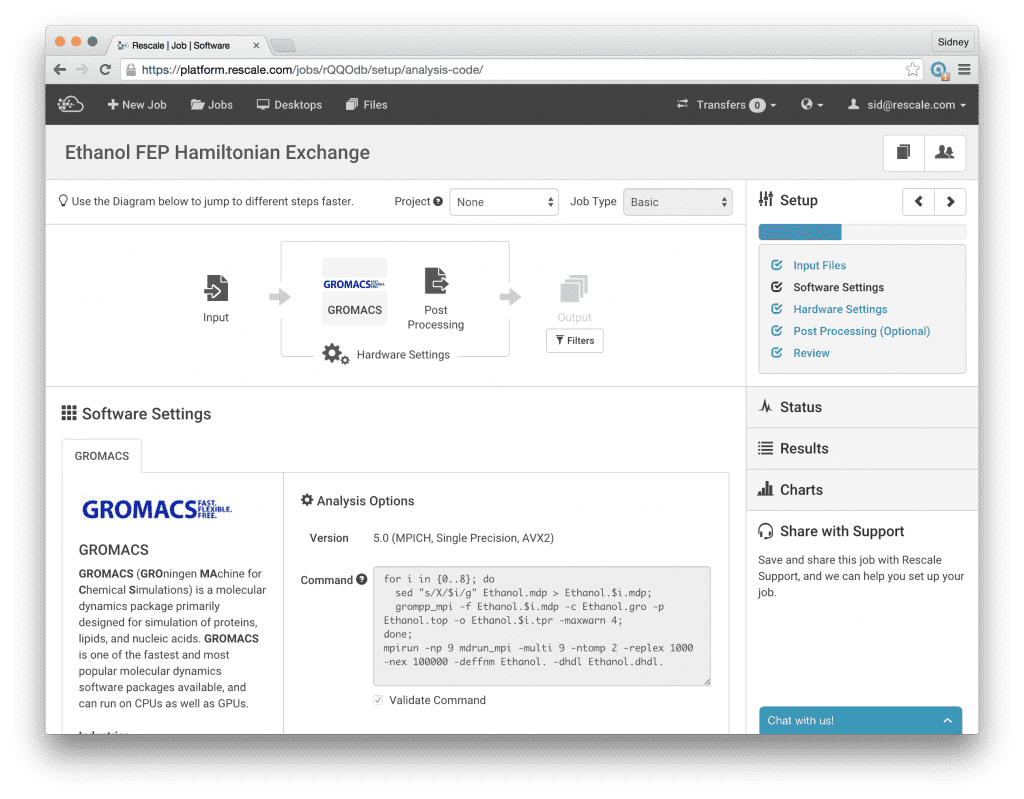# Free Energy Calculations on the Rescale PlatformWithin the life sciences industry, one of the most important simulation methods for developing a new drug is free energy perturbation (FEP), which is a particular method in the class of free energy calculations. In simplified terms, the objective of free energy calculations is to compute the free energy difference between two different chemical states A and B by alchemically transforming state A into state B over the course of several intermediate non-physical chemical states, denoted by lambda. There are several methods available to calculate free energies, including slow growth, thermodynamic integration (TI), and free energy perturbation (FEP); the reason FEP has become a popular method for computing free energies is because of it’s inherent scaling properties which makes it particularly amenable to running in a high performance environment. There are excellent online resources which cover the theory of free energy calculations, so I will not go into more details here, other than to say that the fact that the lambda windows are independent from each other allows us to run multiple simulations in parallel. In practical terms, this means that we can use the Rescale platform to create a compute cluster and divide the work of calculating the free energy into M independent simulations, each with a given value of lambda. To increase the sampling efficiency, we can also couple these independent simulations using a method called Hamiltonian Replica Exchange if the software package we choose to use supports this method.
To demonstrate how easy it is to run these simulations on Rescale, I will take an example from Alchemistry.org for the absolute solvation free energy of Ethanol calculated using GROMACS. In this example, the model has already been built and equilibrated for us so we don’t need to do anything further in regards to model building. The topology file Ethanol.top contains the definitions of the molecules while the coordinate file Ethanol.gro contains the equilibrated 3-dimensional coordinates of the atoms involved in the system. We will use both of these files as they are, without further changes. Moreover, the run input configuration file Ethanol.mdp includes a section for the settings necessary to calculate the free energy using FEP.

```; Free energy parameters
free-energy               = yes
; Which intermediate state are we simulating?
init-lambda-state        = X
; What are the values of lambda at the intermediate states?
coul-lambdas             = 0.0 0.2 0.5 1.0 1.0 1.0 1.0 1.0 1.0
vdw-lambdas              = 0.0 0.0 0.0 0.0 0.2 0.4 0.6 0.8 1.0
; This makes sure we print out the differences in Hamiltonians between all states, and not just the neighboring states
calc-lambda-neighbors = -1
; We are doing free energies with the ethanol molecule alone
couple-moltype           = Ethanol```

According to the settings given in this file, we see that nine intermediate states have been defined using the ‘coul-lambdas’ and ‘vdw-lambdas’ keywords. A given lamdba intermediate state is referenced as a (coul-lambdasi, vdw_lambdasi) pair; therefore, the lengths of each of these arrays must be the same, otherwise, an error will occur. We will run nine separate simulations, one for each intermediate state defined above. The specific lambda value for a given simulation is specified with the ‘init-lambda-state’ keyword and is an integer between 0 and 8. The only work we need to do is write a simple script that will generate the input configuration file for each of the lambda values; this can be done directly within Rescale when setting up a new job, which is discussed further below.
A few comments may be helpful on the other keywords given in the configuration file. First, the ‘free-energy = yes’ keyword tells the simulation engine that we are doing a free energy calculation, and the ‘couple-moltype = Ethanol’ keyword specifies that the ethanol molecule is the only object that will be transformed. In this case, because of the way the ethanol molecule is defined in the topology file, the whole molecule is transformed from a fully interacting molecule to a ghost particle which no longer interacts with the rest of the system. Secondly, the ‘calc-lambda-neighbors = -1’ keyword tells GROMACS to calculate the energy difference between the reference intermediate state and all other intermediate states. This keyword needs to be set in this way in order to do the Multi-state Bennett Acceptance Ratio analysis method.
With this background, let’s set up and run this example calculation on Rescale. First, upload the three input files. I have tarred them together with all three files in the top level directory for simplicity.Next, click Software Settings and select Gromacs. Choose version 5.0 (MPICH, Single Precision, AVX2) and write the command script as shown in the screenshot below to run the calculations. This script loops over the lambda values from 0 to 8, generating a new run input configuration file for each lambda value. The sed command replaces the ‘X’ in the Ethanol.mdp template file with the corresponding value for lambda and saves the new input file with the lambda value included in the filename, e.g. Ethanol.4.mdp. Then we continue the normal GROMACS workflow by calling grompp_mpi to generate the input structure file Ethanol.4.tpr. Finally, we give the command to run the Hamiltonian Replica Exchange simulation:

`mpirun -np 9 mdrun_mpi -multi 9 -ntomp 2 -replex 1000 -nex 100000 -deffnm Ethanol. -dhdl Ethanol.dhdl.`